### 绘制一张图片

``````ctx.drawImage(image, sx, sy, sWidth, sHeight, dx, dy, dWidth, dHeight);
``````

`drawImage` 它可以接受九个参数(不一定全部传入)

• `image`: 它可以是一个 图片的 Image 元素，或者 Video ，Canvas 元素。

• `dx` 表示在画布 x 轴的坐标值

• `dy` 表示在画布 y 轴的坐标值

• `dWidth` 表示在画布绘制的长度

• `dHeight` 表示在画布绘制的高度

• `sx` 表示在画布所绘制图片本身的 x轴 距离

• `sy` 表示在画布所绘制图片本身的 y轴 距离

• `sWidth` 表示所绘制图片的宽度范围，一般默认是图片的完整大小，你也可以将图片的某一部分开始绘制。

• `sHeight` 表示所绘制图片的高度范围，一般默认是图片的完整大小，你也可以将图片的某一部分开始绘制。### 旋转图片

rotate 只支持传入角度的参数 `angle` ，它表示传入的弧度值，比如我们如果需要选择90度，我们需要将其转换为弧度值

``````const angle = 90 * Math.PI / 180;
ctx.rotate(angle);
``````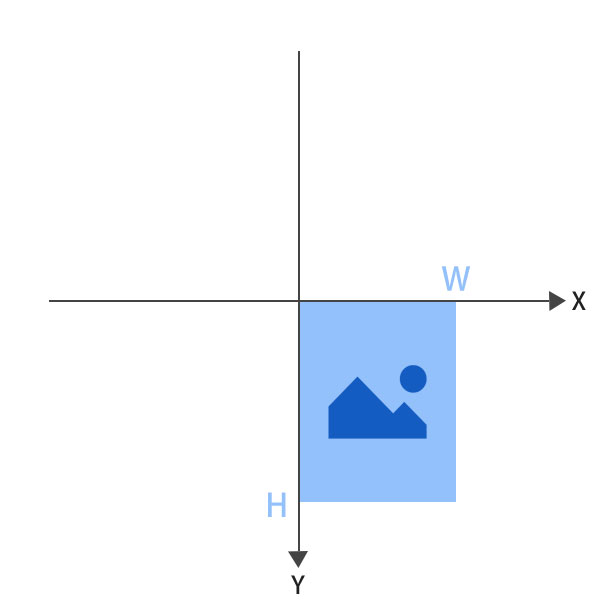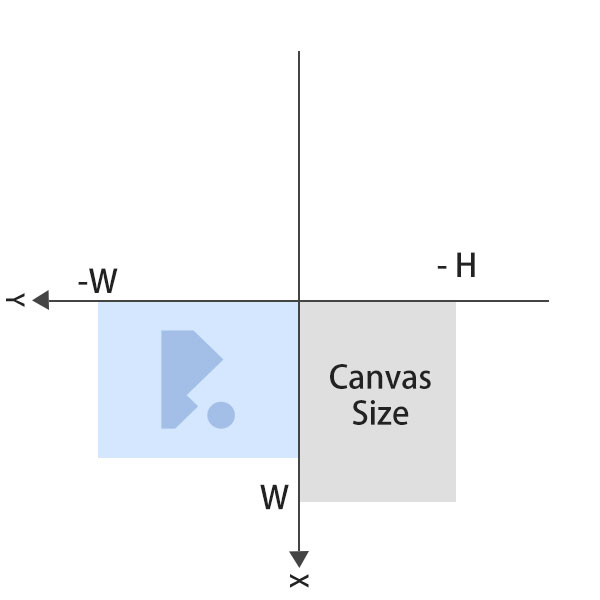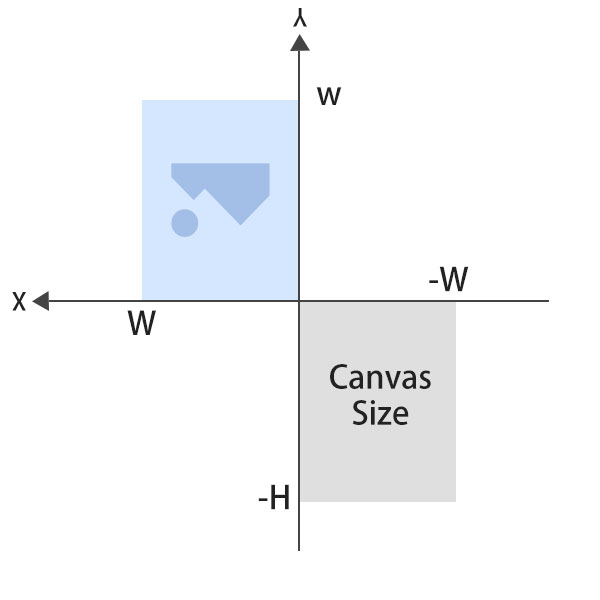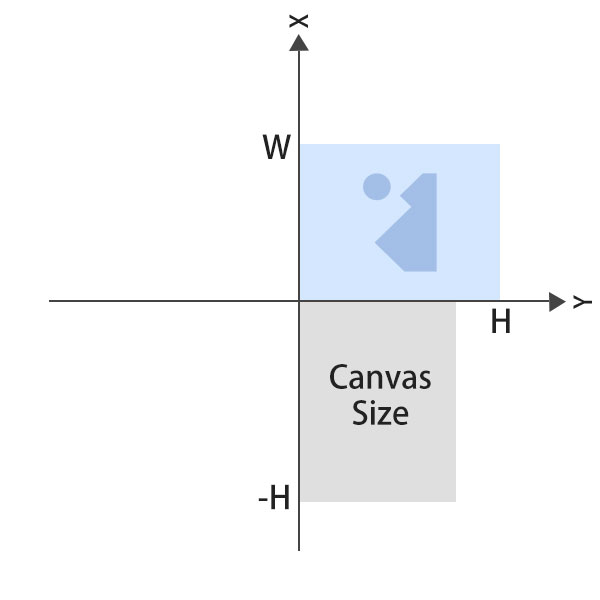``````/**
* compress image
* reference https://github.com/brunobar79/J-I-C
**/

export default {
// .... some other methods

_getImageType(str) {
let mimeType = 'image/jpeg';
const outputType = str.match(/(image\/[\w]+)\.*/);
if (typeof outputType !== 'undefined'){
mimeType = outputType;
}
return mimeType;
},

rotate(src, degrees, callback) {
let w = image.naturalWidth;
let h = image.naturalHeight;
const canvasWidth = Math.max(w, h);
let cvs = this._getCanvas(w, h);
let ctx = cvs.getContext('2d');
ctx.save();
let x = 0;
degrees %= 360;
if (degrees === 0) {
return callback(src, w, h);
}
let y = 0;
if ((degrees % 180) !== 0) {

if (degrees === -90 || degrees === 270) {
x = -h;
} else {
y = -w;
}
const c = w;
w = h;
h = c;
cvs.width = w;
cvs.height = h;
} else {
x = -w;
y = -h;
}
ctx.rotate(degrees * (Math.PI / 180));
ctx.drawImage(image, x, y);
ctx.restore();
const mimeType = this._getImageType(image.src);
const data = cvs.toDataURL(mimeType, 1);
callback(data, w, h);
cvs = null;
ctx = null;
});
},

const image = new Image();
image.src = data;
callback(image);
};
image.onerror = function () {
console.log('Error: image error!');
};
},

_getCanvas(width, height) {
const canvas = document.createElement('canvas');
canvas.width = width;
canvas.height = height;
return canvas;
},

};

``````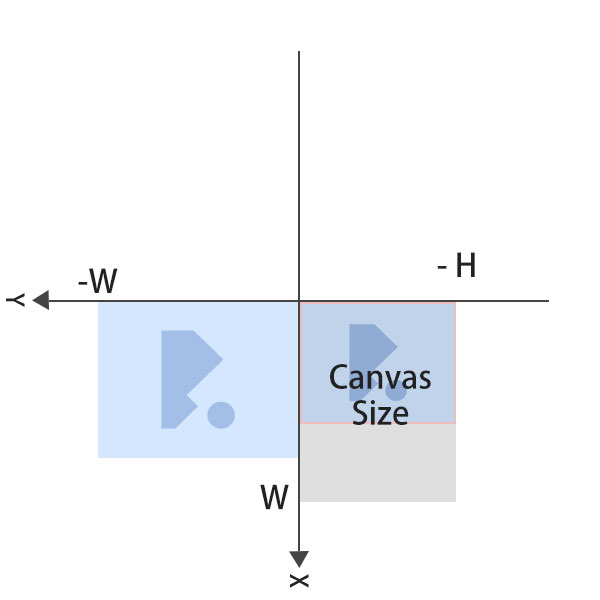`````` const canvasWidth = Math.max(w, h);
const cvs = this._getCanvas(canvasWidth, canvasWidth);
const ctx = cvs.getContext('2d');
``````

``````ctx.translate(canvasWidth / 2, canvasWidth / 2);
``````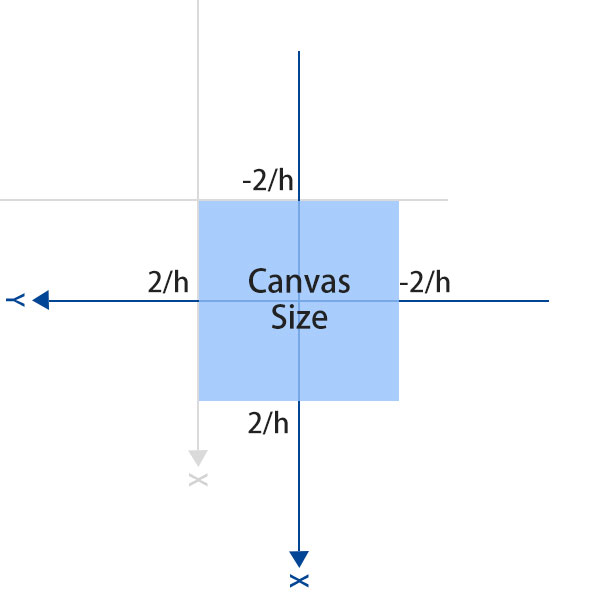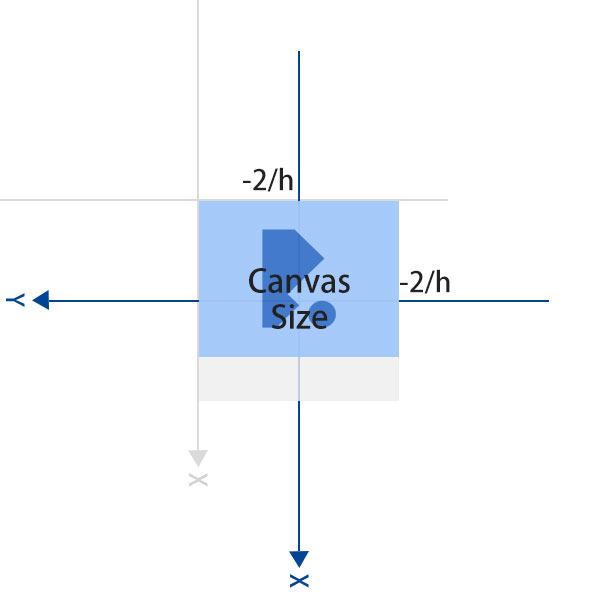``````x = - canvasWidth/2;
y = canvasWidth/2 - h;
``````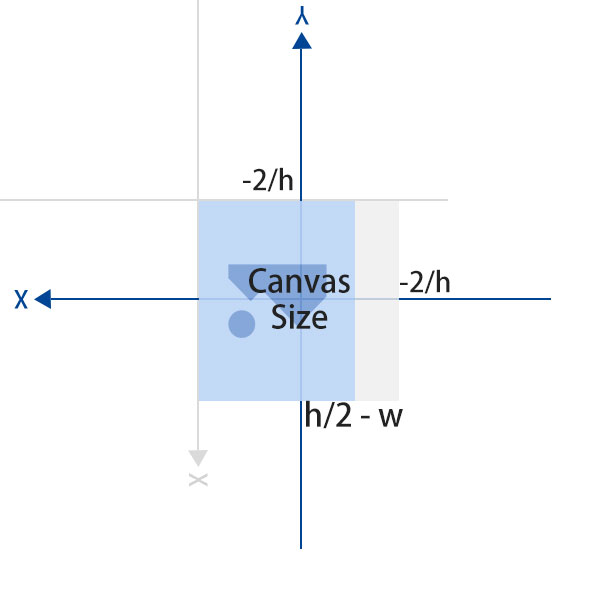``````x = canvasWidth / 2 - h;
y = canvasWidth / 2 - w;
``````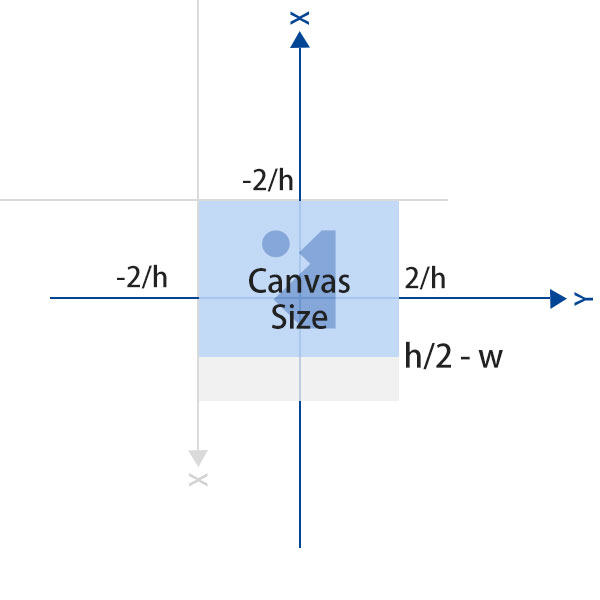``````x = -canvasWidth / 2;
y = canvasWidth / 2 - w;
``````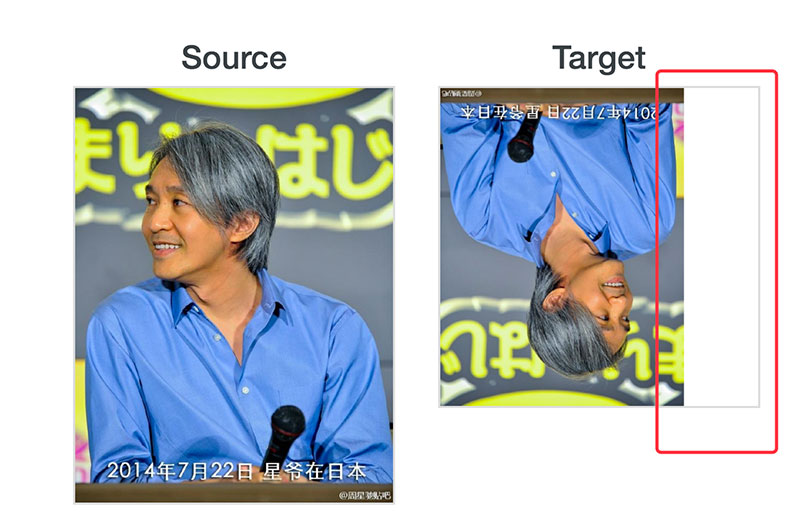``````var cvs2 = _this._getCanvas(w, h);
var ctx2 = cvs2.getContext('2d');
ctx2.drawImage(cvs, 0, 0, w, h, 0, 0, w, h);
var mimeType = _this._getImageType(image.src);
var data = cvs2.toDataURL(mimeType, 1);
``````

Demo

Code

### 扩展阅读

You Can Speak "Hi" to Me in Those Ways